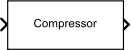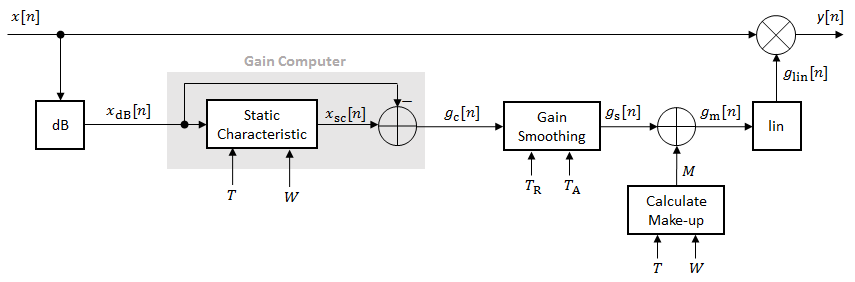# Compressor

Dynamic range compressor

• Library:
• Audio Toolbox / Dynamic Range Control

•## Description

The Compressor block performs dynamic range compression independently across each input channel. Dynamic range compression attenuates the volume of loud sounds that cross a given threshold. The block uses specified attack and release times to achieve a smooth applied gain curve.

## Ports

### Input

expand all

• Matrix input –– Each column of the input is treated as an independent channel.

• 1-D vector input –– The input is treated as a single channel.

This port is unnamed unless you specify additional input ports.

Data Types: `single` | `double`

#### Dependencies

To enable this port, select Specify from input port for the Threshold (dB) parameter.

Data Types: `single` | `double`

#### Dependencies

To enable this port, select Specify from input port for the Ratio parameter.

Data Types: `single` | `double`

#### Dependencies

To enable this port, select Specify from input port for the Knee width (dB) parameter.

Data Types: `single` | `double`

#### Dependencies

To enable this port, select Specify from input port for the Attack time (s) parameter.

Data Types: `single` | `double`

#### Dependencies

To enable this port, select Specify from input port for the Release time (s) parameter.

Data Types: `single` | `double`

### Output

expand all

The Compressor block outputs a signal with the same data type as the input signal. The size of the output depends on the size of the input:

• Matrix input –– The block outputs a matrix the same size and data type as the input signal.

• 1-D vector input –– The block outputs an N-by-1 matrix (column vector), where N is the number of elements in the 1-D vector.

Data Types: `single` | `double`

#### Dependencies

To enable this port, select the Output gain (dB) parameter.

Data Types: `single` | `double`

## Parameters

expand all

If a parameter is listed as tunable, then you can change its value during simulation.

Main Tab

Operation threshold is the level above which gain is applied to the input signal.

To specify Threshold (dB) from an input port, select Specify from input port for the parameter.

Tunable: Yes

Compression ratio is the input/output ratio for signals that overshoot the operation threshold.

Assuming a hard knee characteristic and a steady-state input such that x[n] dB > Threshold (dB), the compression ratio is defined as $R=\frac{\left(x\left[n\right]-T\right)}{\left(y\left[n\right]-T\right)}$, where

• R is the compression ratio.

• x[n] is the input signal in dB.

• y[n] is the output signal in dB.

• T is the threshold in dB.

To specify Ratio from an input port, select Specify from input port for the parameter.

Tunable: Yes

For soft knee characteristics, the transition area is defined by the relation

`$y=x+\frac{\left(\frac{1}{R}-1\right)×{\left(x-T+\frac{W}{2}\right)}^{2}}{\left(2×W\right)}$`

for the range $\left(2×|x-T|\right)\le W$, where

• y is the output level in dB.

• x is the input level in dB.

• R is the compression ratio.

• T is the threshold in dB.

• W is the knee width in dB.

To specify Knee width (dB) from an input port, select Specify from input port for the parameter.

Tunable: Yes

The plot is updated automatically when parameters of the Compressor block change.

Tunable: Yes

Attack time is the time the compressor gain takes to rise from 10% to 90% of its final value when the input goes above the threshold. The Attack time (s) parameter smooths the applied gain curve.

To specify Attack time (s) from an input port, select Specify from input port for the parameter.

Tunable: Yes

Release time is the time the compressor gain takes to drop from 90% to 10% of its final value when the input goes below the threshold. The Release time (s) parameter smooths the applied gain curve.

To specify Release time (s) from an input port, select Specify from input port for the parameter.

Tunable: Yes

• `Property` –– Make-up gain is set to the value specified by the Make-up gain (dB) parameter.

• `Auto` –– Make-up gain is applied at the output of the Compressor block such that a steady-state 0 dB input has a 0 dB output.

Tunable: No

Make-up gain compensates for gain lost during compression. It is applied at the output of the Compressor block.

Tunable: Yes

#### Dependencies

To enable this parameter, set the Make-up gain mode parameter to `Property`.

When you select this parameter, the block inherits its sample rate from the input signal. When you clear this parameter, specify the sample rate in the Input sample rate (Hz) parameter.

Tunable: No

Tunable: Yes

#### Dependencies

To enable this parameter, clear the Inherit sample rate from input parameter.

When you select this parameter, an additional output port, G, is added to the block. The G port outputs the gain applied on each input channel in dB.

Tunable: No

When you select this parameter, an additional input port SC is added to the block. The SC port enables dynamic range compression of the input signal x using a separate sidechain signal.

The datatype and (frame) length input to the SC port must be the same as the input to the x port.

The number of channels of the sidechain input must be equal to the number of channels of `x` or be equal to one.

• `Sidechain channel count is equal to one` –– The computed gain, `G`, based on this channel is applied to all channels of `x`.

• ```Sidechain channel count is equal to channel count of ````x` –– The computed gain, `G`, for each sidechain channel is applied to the corresponding channel of `x`.

• `Interpreted execution` –– Simulate model using the MATLAB® interpreter. This option shortens startup time and has a simulation speed comparable to ```Code generation```. In this mode, you can debug the source code of the block.

• `Code generation` –– Simulate model using generated C code. The first time you run a simulation, Simulink® generates C code for the block. The C code is reused for subsequent simulations, as long as the model does not change. This option requires additional startup time, but the speed of the subsequent simulations is comparable to ```Interpreted execution```.

Tunable: No

## Block Characteristics

 Data Types `double` | `single` Direct Feedthrough `no` Multidimensional Signals `no` Variable-Size Signals `yes` Zero-Crossing Detection `no`

## Algorithms

The Compressor block processes a signal frame by frame and element by element.1. The N-point signal, x[n], is converted to decibels:

`${x}_{\text{dB}}\left[n\right]=20×{\mathrm{log}}_{10}|x\left[n\right]|$`

2. xdB[n] passes through the gain computer. The gain computer uses the static compression characteristic of the Compressor block to attenuate gain that is above the threshold.

If you specified a soft knee, the gain computer has the following static characteristic:

`${x}_{\text{sc}}\left({x}_{\text{dB}}\right)=\left\{\begin{array}{cc}{x}_{\text{dB}}& {x}_{\text{dB}}<\left(T-\frac{W}{2}\right)\\ {x}_{\text{dB}}+\frac{\left(\frac{1}{R}-1\right){\left({x}_{\text{dB}}-T+\frac{W}{2}\right)}^{2}}{2W}& \left(T-\frac{W}{2}\right)\le {x}_{\text{dB}}\le \left(T+\frac{W}{2}\right)\\ T+\frac{\left({x}_{\text{dB}}-T\right)}{R}& {x}_{\text{dB}}>\left(T+\frac{W}{2}\right)\end{array}\text{ },$`

where T is the threshold, R is the compression ratio, and W is the knee width.

If you specified a hard knee, the gain computer has the following static characteristic:

`${x}_{\text{sc}}\left({x}_{\text{dB}}\right)=\left\{\begin{array}{cc}{x}_{\text{dB}}& {x}_{\text{dB}}`

3. The computed gain, gc[n], is calculated as

`${g}_{\text{c}}\left[n\right]={x}_{\text{sc}}\left[n\right]-{x}_{\text{dB}}\left[n\right].$`

4. gc[n] is smoothed using specified attack and release time parameters:

`${g}_{\text{s}}\text{[}n\right]=\left\{\begin{array}{cc}{\alpha }_{\text{A}}{g}_{\text{s}}\left[n-1\right]+\left(1-{\alpha }_{\text{A}}\right){g}_{\text{c}}\left[n\right],& {g}_{\text{c}}\left[n\right]\le {g}_{\text{s}}\left[n-1\right]\\ {\alpha }_{\text{R}}{g}_{\text{s}}\text{[}n-1\right]+\left(1-{\alpha }_{\text{R}}\right){g}_{\text{c}}\left[n\right],& {g}_{\text{c}}\left[n\right]>{g}_{\text{s}}\left[n-1\right]\end{array}$`

The attack time coefficient, α A, is calculated as

`${\alpha }_{\text{A}}=\mathrm{exp}\left(\frac{-\mathrm{log}\left(9\right)}{Fs×{T}_{\text{A}}}\right)\text{\hspace{0.17em}}.$`

The release time coefficient, α R, is calculated as

`${\alpha }_{\text{R}}=\mathrm{exp}\left(\frac{-\mathrm{log}\left(9\right)}{Fs×{T}_{\text{R}}}\right)\text{\hspace{0.17em}}.$`

T A is the attack time period, specified by the Attack time (s) parameter. TR is the release time period, specified by the Release time (s) parameter. Fs is the input sampling rate, specified by the Inherit sample rate from input or the Input sample rate (Hz) parameter.

5. If Make-up gain (dB) is set to `Auto`, the make-up gain is calculated as the negative of the computed gain for a 0 dB input:

`$M={-{x}_{\text{sc}}|}_{{x}_{\text{dB}}=0}.$`

Given a steady-state input of 0 dB, this configuration achieves a steady-state output of 0 dB. The make-up gain is determined by the Threshold (dB), Ratio, and Knee width (dB) parameters. It does not depend on the input signal.

6. The make-up gain, M, is added to the smoothed gain, gs[n]:

`${g}_{\text{m}}\left[n\right]={g}_{\text{s}}\left[n\right]+M$`

7. The calculated gain in dB, gdB[n], is translated to a linear domain:

`${g}_{\text{lin}}\left[n\right]={10}^{\left(\frac{{g}_{\text{m}}\left[n\right]}{20}\right)}$`

8. The output of the dynamic range compressor is given as

`$y\left[n\right]=x\left[n\right]×{g}_{\text{lin}}\left[n\right].$`

 Giannoulis, Dimitrios, Michael Massberg, and Joshua D. Reiss. "Digital Dynamic Range Compressor Design –– A Tutorial And Analysis." Journal of Audio Engineering Society. Vol. 60, Issue 6, 2012, pp. 399–408.